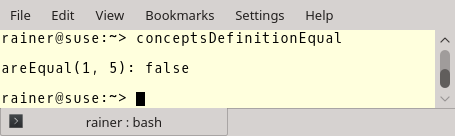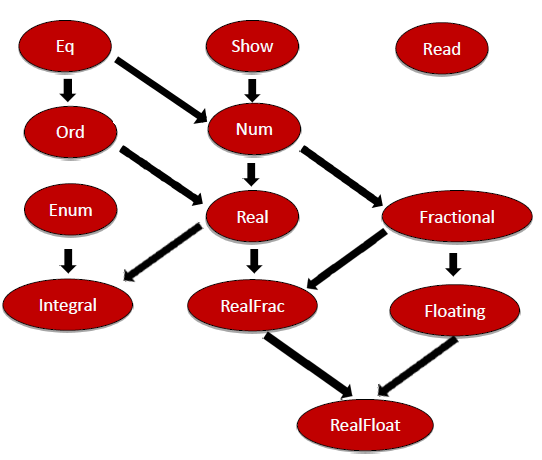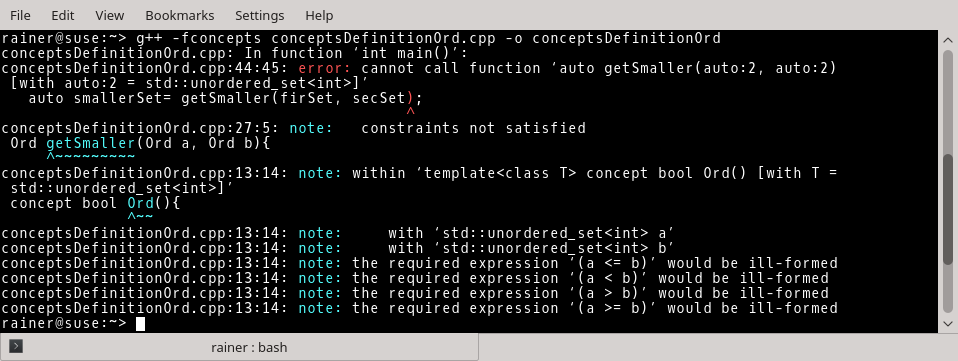# Defining Concepts

I wrote a few posts about using concepts. Concepts are a named set of requirements. Let’s define a few concepts in this post.

A concept can be defined by a function template or by a variable template. A variable template is new with C++14 and declares a family of variables. If you use a function template for your concept, it’s called a function concept; in the second case a variable concept.

## Two forms

```template<typename T>
concept bool Integral = std::is_integral<T>::value;
}

template<typename T>
concept bool Equal(){
return requires(T a, T b) {
{ a == b } -> bool;
{ a != b } -> bool;
};
}
```

Integral is a variable concept and Equal is a functional concept. Both return a boolean.

• The type parameter T fulfills the variable concept Integral if std::is_integral<T>::value returns true.
• The type parameter T fulfills the function concept Equal if there are overloaded operators == and != for T that returns a boolean.

The function concept Equal look very familiar to me. Why? You will see it in a few sentences. But let me first apply the concept.

##Modernes C++ Mentoring

Be part of my mentoring programs:

• "Fundamentals for C++ Professionals" (open)
• "Design Patterns and Architectural Patterns with C++" (open)
• "C++20: Get the Details" (reopens December 2023)
• Do you want to stay informed about my mentoring programs: Subscribe via E-Mail.

 ``` 1 2 3 4 5 6 7 8 9 10 11 12 13 14 15 16 17 18 19 20 21 22 23 24 25 26 27 28 29 30 31 32 33 34 35 36 37 38 39 40 41 42 43 44 45``` ```// conceptsDefintionEqual.cpp #include template concept bool Equal(){ return requires(T a, T b) { { a == b } -> bool; { a != b } -> bool; }; } bool areEqual(Equal a, Equal b){ return a == b; } /* struct WithoutEqual{ bool operator==(const WithoutEqual& other) = delete; }; struct WithoutUnequal{ bool operator!=(const WithoutUnequal& other) = delete; }; */ int main(){ std::cout << std::boolalpha << std::endl; std::cout << "areEqual(1, 5): " << areEqual(1, 5) << std::endl; /* bool res = areEqual(WithoutEqual(), WithoutEqual()); bool res2 = areEqual(WithoutUnequal(), WithoutUnequal()); */ std::cout << std::endl; } ```

I used the concept Equal in the (generic) function areEqual (lines 13 to 15). That’s not so exciting. Here is the output of the function areEqual:What is more interesting is if I use the class WithoutEqual and WithoutUnequal.I set for both the == or respectively the != operator to delete. The compiler complains immediately that both types do not fulfill the concept.Equal look familiar to me. Now, you see why.

## The concept Equal and OrdThis is part of the type hierarchy of Haskell’s type classes. You have a kind of inheritance between the type classes, denoted by arrows. Looking in the left corner at the top, you will see the typeclass Eq. Now I’m curious how the definition of Eq will look like.

```class Eq a where
(==) :: a -> a -> Bool
(/=) :: a -> a -> Bool

template<typename T>
concept bool Equal(){
return requires(T a, T b) {
{ a == b } -> bool;
{ a != b } -> bool;
};
}
```

Let’s have a closer look at Haskell’s typeclass Eq. Eq requires, from its instances, that

• they have equal == and inequal /= operation that returns a Bool.
• both take two arguments (a -> a) of the same type.

Of course, the instances are the concrete types such as Int.

Now, I have two questions in mind if I look at Haskell’s type hierarchy. What is the definition of the typeclass Ord in Haskell, and can we model the inheritance relation in C++?

What is the definition of the typeclass Ord in Haskell?

### Ord

```class Eq a => Ord a where
compare :: a -> a -> Ordering
(<) :: a -> a -> Bool
(<=) :: a -> a -> Bool
(>) :: a -> a -> Bool
(>=) :: a -> a -> Bool
max :: a -> a -> a
```

The most exciting point about the typeclass Ord is the first line of its definition. An instance of the typeclass Ord must already be an instance of the typeclass Eq. Ordering is an enumeration having the values EQ, LT, and GT.

How can we model the concept Ord in C++?

### Eq -> Ord

Of course, we can define the concept Ord by using all requirements of Eq and Ord. But we can do better in C++:

 ``` 0 1 2 3 4 5 6 7 8 9 10 11 12 13 14 15 16 17 18 19 20 21 22 23 24 25 26 27 28 29 30 31 32 33 34 35 36 37 38 39 40 41 42 43 44 45 46 47 48 49``` ```// conceptsDefintionOrd.cpp #include #include template concept bool Equal(){ return requires(T a, T b){ { a == b } -> bool; { a != b } -> bool; }; } template concept bool Ord(){ return requires(T a, T b){ requires Equal(); { a <= b } -> bool; { a < b } -> bool; { a > b } -> bool; { a >= b } -> bool; }; } bool areEqual(Equal a, Equal b){ return a == b; } Ord getSmaller(Ord a, Ord b){ return (a < b) ? a : b; } int main(){ std::cout << std::boolalpha << std::endl; std::cout << "areEqual(1, 5): " << areEqual(1, 5) << std::endl; std::cout << "getSmaller(1, 5): " << getSmaller(1, 5) << std::endl; std::unordered_set firSet{1, 2, 3, 4, 5}; std::unordered_set secSet{5, 4, 3, 2, 1}; std::cout << "areEqual(firSet, secSet): " << areEqual(firSet, secSet) << std::endl; // auto smallerSet= getSmaller(firSet, secSet); std::cout << std::endl; } ```

To simplify my job, I ignored the requirements compare and max in the concept Ord. The critical point about the concept is the line requires Equal<T>(). Here I require that the type parameter T fulfill the requirement Equal. If I use more requirements, such as in the definition of the concept Equal, each requirement from top to bottom will be checked. That will be done in a short-circuiting evaluation. So the first requirement returning false will end the process.

Equality and inequality are defined for the data types int and std::unordered_set. Therefore, the output should not surprise you.That will change dramatically if I use line 44 because the smaller/bigger operators are not defined for std::unordered_set.## What’s next?

I wrote a few articles for the German Linux-Magazin and iX about C++17. One of my blog readers asked me if they are available in English. I answered no. But I promised him to write about C++17 in my next post.

Thanks a lot to my Patreon Supporters: Matt Braun, Roman Postanciuc, Tobias Zindl, G Prvulovic, Reinhold Dröge, Abernitzke, Frank Grimm, Sakib, Broeserl, António Pina, Sergey Agafyin, Андрей Бурмистров, Jake, GS, Lawton Shoemake, Jozo Leko, John Breland, Venkat Nandam, Jose Francisco, Douglas Tinkham, Kuchlong Kuchlong, Robert Blanch, Truels Wissneth, Kris Kafka, Mario Luoni, Friedrich Huber, lennonli, Pramod Tikare Muralidhara, Peter Ware, Daniel Hufschläger, Alessandro Pezzato, Bob Perry, Satish Vangipuram, Andi Ireland, Richard Ohnemus, Michael Dunsky, Leo Goodstadt, John Wiederhirn, Yacob Cohen-Arazi, Florian Tischler, Robin Furness, Michael Young, Holger Detering, Bernd Mühlhaus, Matthieu Bolt, Stephen Kelley, Kyle Dean, Tusar Palauri, Dmitry Farberov, Juan Dent, George Liao, Daniel Ceperley, Jon T Hess, Stephen Totten, Wolfgang Fütterer, Matthias Grün, Phillip Diekmann, Ben Atakora, Ann Shatoff, Rob North, Bhavith C Achar, and Marco Parri Empoli.

Thanks, in particular, to Jon Hess, Lakshman, Christian Wittenhorst, Sherhy Pyton, Dendi Suhubdy, Sudhakar Belagurusamy, Richard Sargeant, Rusty Fleming, John Nebel, Mipko, Alicja Kaminska, Slavko Radman, and David Poole.

 My special thanks to EmbarcaderoMy special thanks to PVS-StudioMy special thanks to Tipi.buildMy special thanks to Take Up Code## Seminars

I’m happy to give online seminars or face-to-face seminars worldwide. Please call me if you have any questions.

### Standard Seminars (English/German)

Here is a compilation of my standard seminars. These seminars are only meant to give you a first orientation.

• C++ – The Core Language
• C++ – The Standard Library
• C++ – Compact
• C++11 and C++14
• Concurrency with Modern C++
• Design Pattern and Architectural Pattern with C++
• Embedded Programming with Modern C++
• Generic Programming (Templates) with C++

#### New

• Clean Code with Modern C++
• C++20

### Contact Me

Modernes C++ Mentoring,Tags:
0 replies